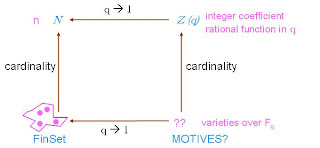occasional meanderings in physics' brave new world

## Friday, December 07, 2007

Whoa! I wasn't expecting it that soon. Motives appear already, at least conjecturally, in John Baez's lecture 6!In the diagram the vertical arrows are the decategorification of either sets or projective spaces. An isomorphism class of $n$ element sets is mapped to the number $n$. Natural numbers become $q$-numbers in the case of spaces, which is to say rational functions in the parameter $q$ corresponding to the number of elements in the finite field, or secretly really polynomials with integer coefficients. But what replaces the category of finite sets? There is more structure to the projective spaces, and we also want to understand the bottom arrow, which considers a set as a space over a one element field.

This is a rather delicate mathematical question. Baez mentions a recent paper by Durov (with lots of stuff on monads) about the idea of a one element field. When we understand this properly, do we find motives? Now, that is the question.

The plane of a (finite) field $F_{q}^{2}$ is the $q$-analogue of a two element set, which plays an important role in the Boolean topos Set, namely as the subobject classifier. The vector space version of this is commonly known as a qubit. Somehow the reason that a one element field $F_1$ doesn't usually make sense is because the logical 0 and 1 are not distinct. Since $q$ is, in the first instance, just a natural number (= $p^{k}$ for some prime $p$), we can ask ourselves first what it means to collapse a finite plane to a one element field. For the topos Set, this would amount to turning the whole category into the trivial category 1, since there is no way to distinguish a subset of a set $S$ from its complement and all sets have effectively only one element. Now this one element set is like a basis for spaces over $F_1$. But the map that takes a basis to a space is just the functor 1 $\rightarrow$ Set, which picks out a set!

But this doesn't sound right. Maybe what we need here is not the trivial 1-category, or a one point 0-category (set), but rather a -1-category. This idea always lurks in the operad heirarchy, where the left hand side of the table starts with the single leaf tree, despite the fact that a point is actually a two leaf tree.

Anyway, think of a finite set $S$ lying at the endpoints of the unit vectors in a vector space. The empty set at the origin is the smallest piece of the power set of $S$, and the one element subsets are the next smallest pieces. The power set fills out a cube of dimension $|S|$. Since the field in question is $F_1$ there is no extent to the axes. Only the elements of $S$ really exist.nige said...

Gasp ... how much study is needed to understand this properly? Did you do an undergraduate degree in mathematics before starting your maths PhD? I understand very little (very few bits and pieces) in this area advanced part of mathematical physics. I have to keep looking up technical terms like decategorification and isomorphism. I even have to refresh my memory on stuff I should recall like projective spaces. Is "motive" a special mathematical term as used here, or something to do with physical connections? (Sorry if this turns out to be so elementary that it appears a foolish or time-wasting question.)

It must be great to know this sort of advanced mathematics and understand what Dr Baez is saying.

December 07, 2007 11:22 PMKea said...

Gasp ... how much study is needed to understand this properly?

More than is humanly possible. Yes, motive is a technical term. And I spent most of the 1990s studying mathematics, after my undergrad degree in physics in the late 1980s, for which I also did a fair bit of mathematics, after a childhood of loving mathematics. And after all that, I know next to nothing.

December 08, 2007 7:59 AMnige said...

Well, I hope that the connection between this mathematics and the physics of nature pans out. It is nice that you put in a lot of hard work over a decade to become expert in this, without receiving big research grants or hype. Even if I was really able to do proper high-level mathematics, I probably wouldn't be able to put up with mainstream fashion and consensus in academia (string theory). It would drive most people out.

What interested me in particular was that you linked to a page by John Baez and James Dolan about the Quantum Gravity Seminar on geometric representation theory.

December 08, 2007 1:17 PM